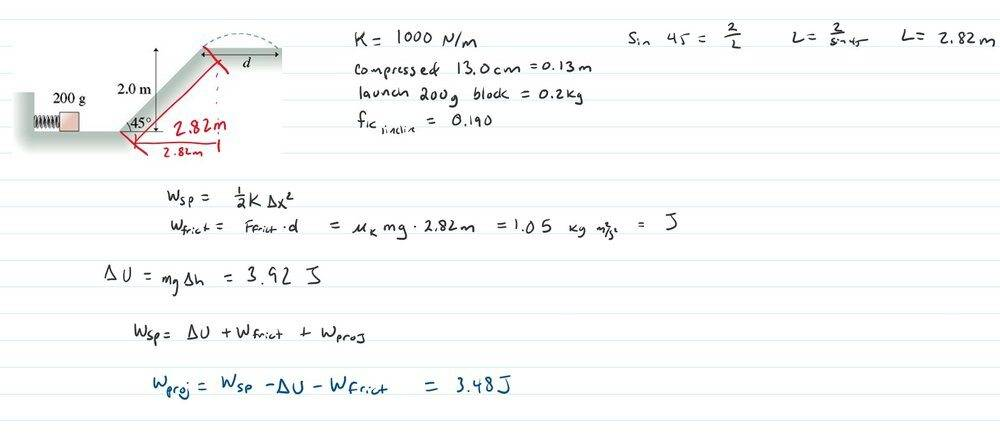# Spring launches a block up a ramp -- How far does it go?

Homework Statement:
The spring in the figure has a spring constant of 1000 N/m . It is compressed 13.0 cm , then launches a 200 g block. The horizontal surface is frictionless, but the block's coefficient of kinetic friction on the incline is 0.190.
Relevant Equations:
Wsp=1/2k(delta x)^2
Wfrict=mu(k)*mgd
Delta U=mg(delta y)
I think I have all the pieces here, and am able to solve for a work through the air. But I have a power output, and don't know how to isolate it to find the distance.Last edited:

I see now I had an error with my potential energy. Height is 2m not 2.82m I like originally calculated. So at the top of the ramp, it still has 3.48 J of projectile motion. I don't know how to convert that to the velocity/acceleration to find the length d.

haruspex
Homework Helper
Gold Member
2020 Award
Check your equation for the frictional force.

•jskrzypi
Lnewqban
Gold Member
Could you draw a FBD for an instant in which the block is climbing the ramp?
Then, compare the forces and reactions with what you have learned about kinetic friction.

The potential energy of the spring becomes kinetic energy, which means there is velocity of the block at the bottom of the incline.
Some of the original kinetic energy is consumed by the friction of the slope.
In order to fly, the block will need to have enough velocity at the moment it reaches the top of the slope.

The flight time involves new transformations of energy: from top of slope kinetic to maximun reached height gravitational potential to crashing down kinetic energy (disregarding any friction with air).

•jskrzypi
So I believe I figured out my error with the friction [needed to multiply by cos 45]. That still leaves me with 3.785 J of projectile work. If K=1/2 mv^2, then v=6.15 m/s launched at a 45 degree angle.

I just found the equation for range...R=2vx*vy/g. Came out to the correct answer of 3.86m.

Thank you both for the assistance.

•Lnewqban